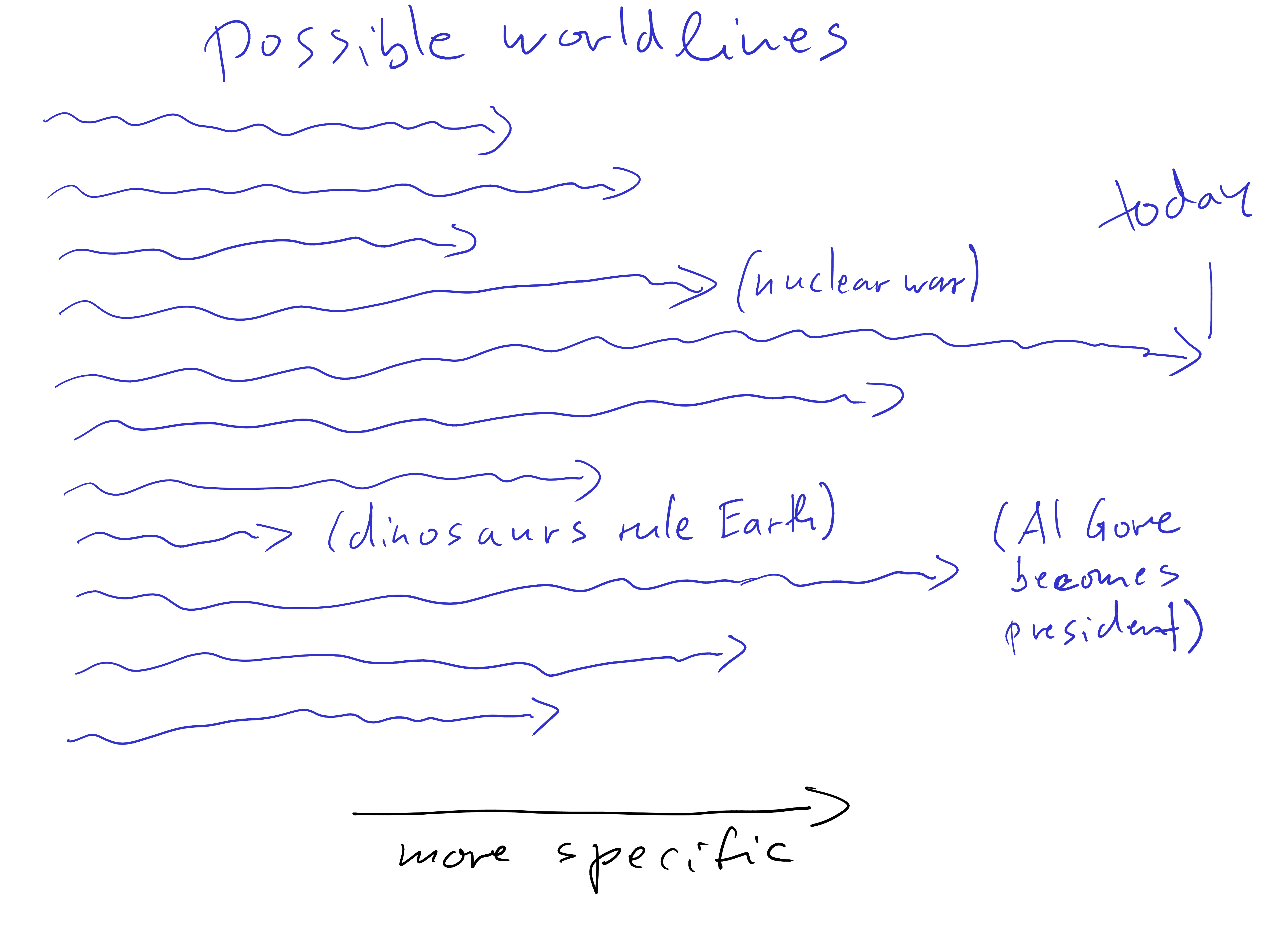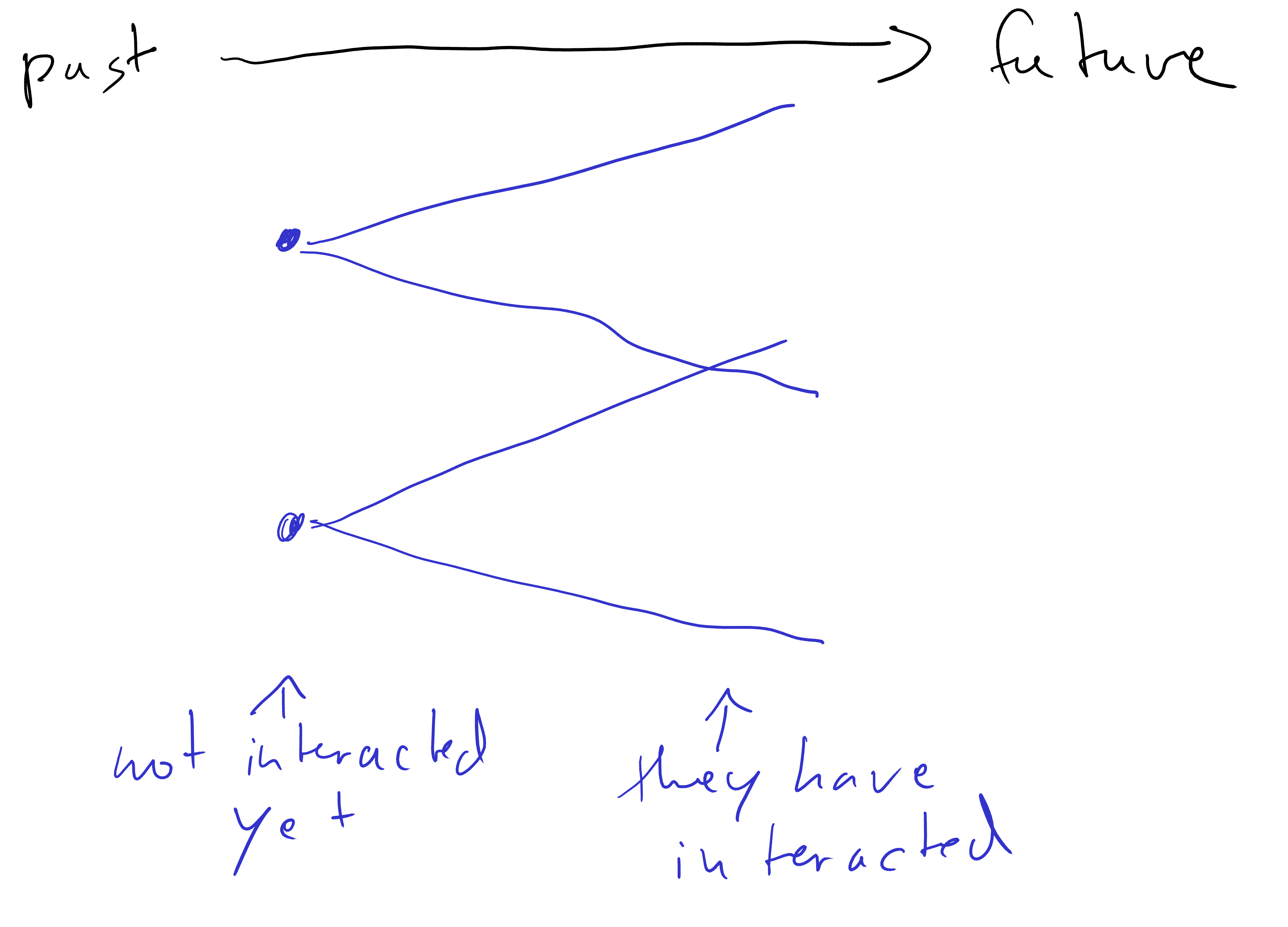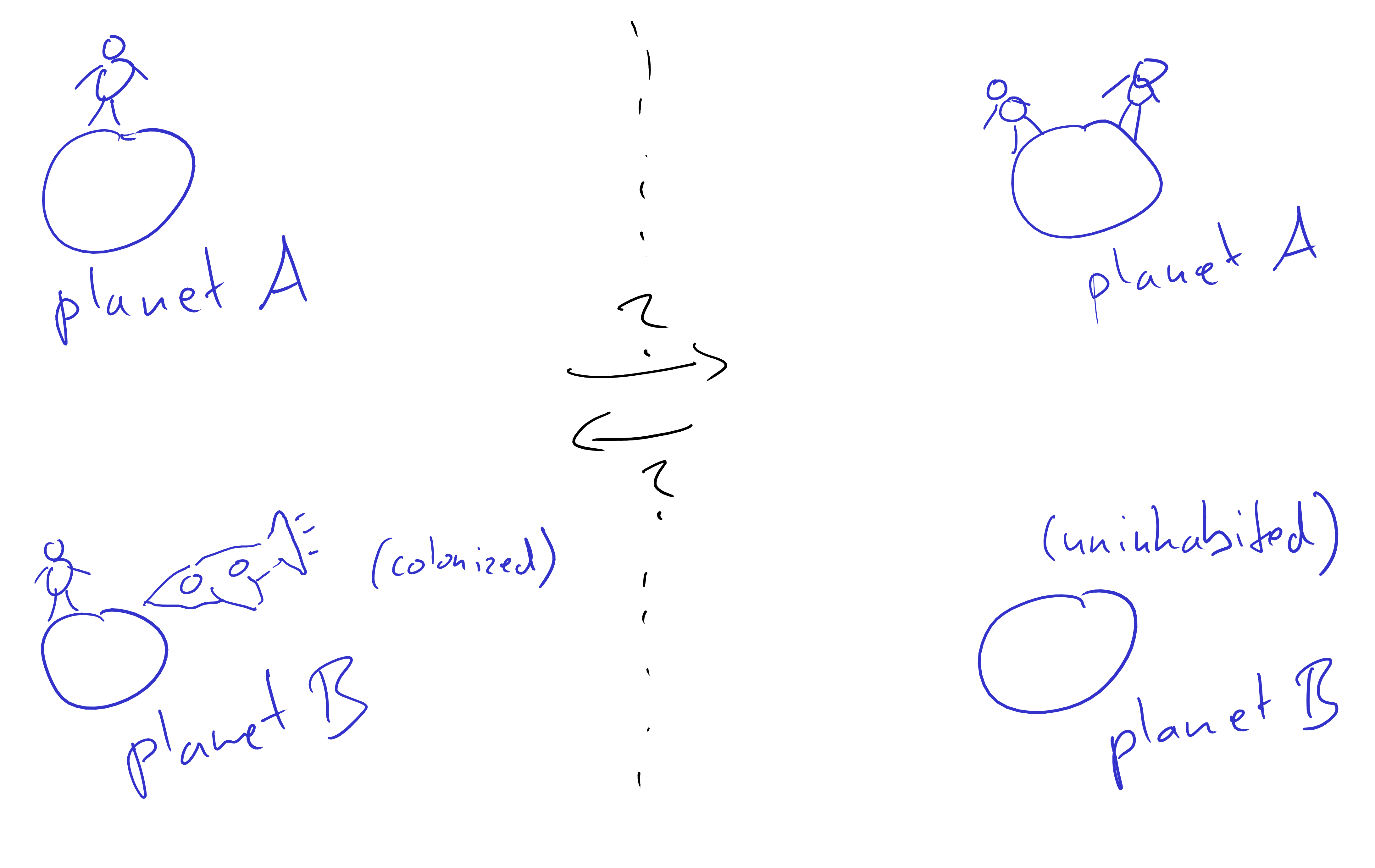Statistical Time or Timeless Causality

## Statistical Time or Timeless Causality

Thomas Kehrenberg

21st April 2022

### Refresher on Bayesian networks

Bayesian networks are just visual representations of independencies.

$$P(x_1, ..., x_n)=\prod_i P(x_i|pa(x_i))$$

in this case:

$$P(A, B, C, D, E)=P(A)P(B|A)P(C|B)P(D|B)P(E|A,C)$$

No.

### These two imply the same factorization

$$P(A,B)=P(A)P(B|A)$$

$$P(A,B)=P(B)P(A|B)$$

So: meaningless arrows for now

### With 3 nodes, it’s also not guaranteed.

$$P(A, B, C) \\= P(C|A)P(B|A)P(A)$$

so: $$P(C|A,B)=P(C|A)$$

so: $$B\perp C|A$$

$$P(A, B, C)\\=P(C|A)P(A|B)P(B)$$

so: $$P(C|A,B)=P(C|A)$$

so: $$B\perp C|A$$

### But with a collider we can!

implied factorization: $$P(A, B, C) = P(C|A, B)P(A)P(B)$$

so: $$P(A|B)=P(A)$$

so: $$A\perp B$$

(but: $$A\not\perp C\quad B\not\perp C\quad A\not\perp C|B\quad B\not\perp C|A\quad A\not\perp B|C$$)

### There are 25 possible DAGs with 3 nodes

wanted: $$A\perp B$$ (and no other independence)wanted: $$A\perp B$$ (and no other independence)

### Do the arrows in colliders represent causality?

Potentially.

But maybe 3 nodes is not enough to define time.

### We need 4 nodes

$$A\perp B$$

$$X\perp Y|A,B$$

(there are 543 DAGs with 4 nodes...)

### Try it out with this Markov chain process

$$G_t = \alpha G_{t-1} + \beta H_{t-1} + \xi_t$$

$$H_t = \gamma G_{t-1} + \delta H_{t-1} + \eta_t$$

$$\xi_t$$, $$\eta_t$$: iid noise (very important)

You will be able to uniquely recover the structure of the chain.

### Claim:

if you have variables $$A$$, $$B$$, $$X$$, and $$Y$$ that are consistent with this DAG,

then $$A$$ and $$B$$ happened before $$X$$ and $$Y$$:

$$A, B <_T X, Y$$

(this is Statistical Time)

### Intuition for why there is an asymmetry between past and future

Assume stochastic world• Another insight is that things become more entangled as time goes on.
• In the past, there might be points that have not causally interacted yet.
• But this gets rarer and rarer as time goes on.$$X\perp Y|A,B$$

$$A\not\perp B|X,Y$$

• from the perspective of the past, the future hasn’t become entangled yet
• from the perspective of the future, the past is very entangled

### Examples

of the form:

we know the true time and check whether statistical time agrees with it

• $$A$$: was there an earthquake?
• $$B$$: did a burglar come to my house?
• $$X$$: is the backyard window broken?
• $$Y$$: is the dog in the front yard barking? (who cannot hear or see the backyard window)

$$A\perp B$$ ?

$$A\not\perp B|X,Y$$ ?

$$X\perp Y|A,B$$ ?

$$X\not\perp Y$$ ?